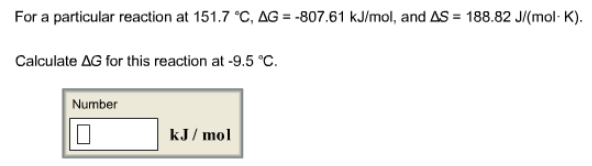# Problem: For a particular reaction at 15 1.7°C, ΔG = - 80761 kJ/mol, and ΔS = 188.82 J/(mol • K). Calculate ΔG for this reaction at -9.5°C.

###### FREE Expert Solution
80% (243 ratings)###### Problem Details

For a particular reaction at 15 1.7°C, ΔG = - 80761 kJ/mol, and ΔS = 188.82 J/(mol • K).

Calculate ΔG for this reaction at -9.5°C.